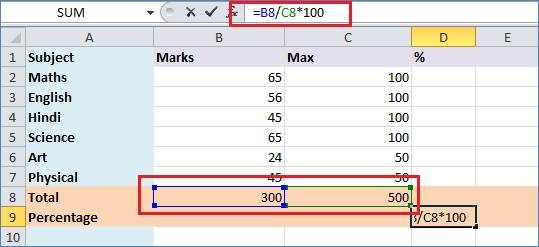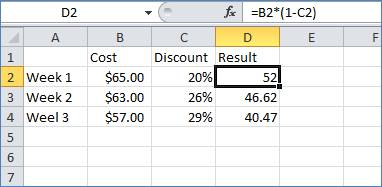In this article I have described to calculate percentages between different cell values. For example compute a sales tax, or determine a percent change in sales between two fiscal quarters etc. There are several ways to calculate percentages.

Percentages are calculated by using the following equation:

amount/total = percentage

Note: Percentage is in decimal format.

For demonstration I have get percentage of get marks as below image data.

=B8/C8*100Now, let's say that you want to reduce a particular amount by %, like when you're trying to apply a discount. Here's a formula that would get you that result. (Think of the 1 in the formula below as a stand-in for 100%.)Modified On Mar-31-2019 07:30:55 PM